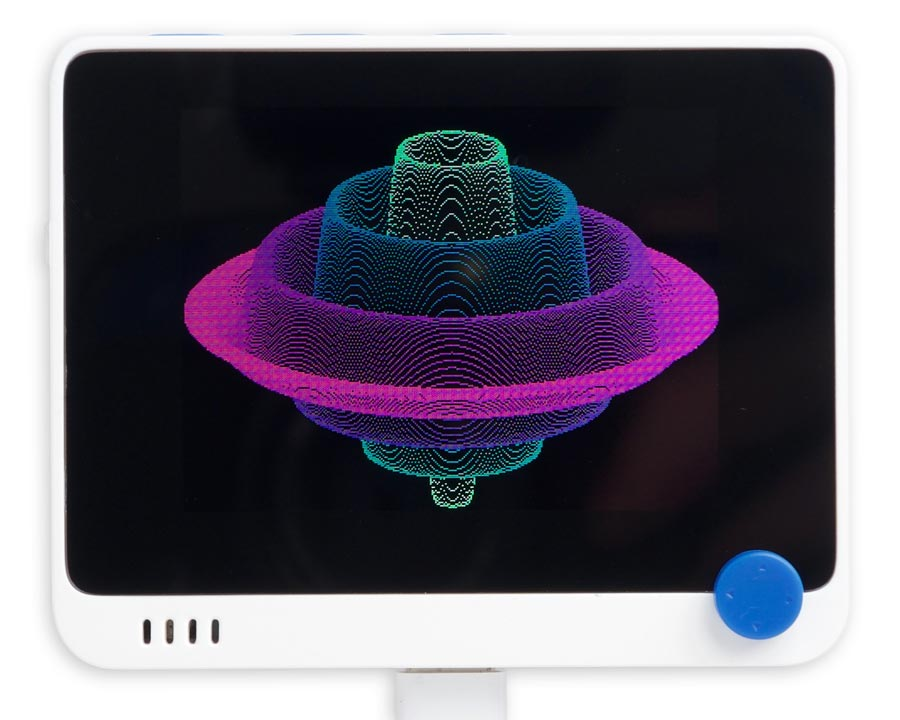## Surface of rotation

This program plots a surface of rotation, with hidden line removal to hide lines behind the surface. It can be run on any display supported by the Graphics extensions.

Here it is running on the Seeed Studio Wio Terminal:### The program

Here's the rotation program:

```(defun rgb (r g b)
(logior (ash (logand r #xf8) 8) (ash (logand g #xfc) 3) (ash b -3)))

(defun rotation (width height fun)
(let ((w2 (truncate width 2))
(h2 (truncate height 2))
m n)
(fill-screen)
(dotimes (x w2)
(let ((p (truncate (sqrt (- (* w2 w2) (* x x))))))
(dotimes (v (* 2 p))
(let* ((z (- v p))
(r (/ (sqrt (+ (* x x) (* z z))) w2))
(q (funcall #'fun r))
(y (round (+ (/ z 3) (* q h2))))
(c (rgb (truncate (* r 255)) (truncate (* (- 1 r) 255)) 128)))
(when
(cond
((zerop v) (setq m y) (setq n y))
((> y m) (setq m y))
((< y n) (setq n y))
(t nil))
(draw-pixel (- w2 x) (- h2 y) c)
(draw-pixel (+ w2 x) (- h2 y) c))))))))```

#### Calling the rotation program

Call the program with:

`(rotation width height function)`

where the first two parameters specify the width and height of the display, and the third parameter is a function to be plotted.

The function should be a function of one parameter, the radius, which goes from 0 to 1. It should return a value between 1 and -1.

#### An example function

For example, the function shown at the start of the article is:

`(defun sinfun (r) (* (- r 1) (sin (* r 24))))`

and you would plot this with:

`(rotation 320 240 sinfun)`

This function defines the equation:

q = (r - 1) * sin(24 * r)

where the sine function gives the rippled effect, and the (r - 1) causes the ripples to die away towards the edge of the curve. The colour of the lines changes as a function of r.

#### Another example

Here's another function that gives a nice plot:

`(defun sqfun (r) (+ (* 2.5 (- 1 r) (- 1 r)) (* 2 r r 0.7) -1.5))`

Here’s the whole program in a single file: Surface of rotation program.